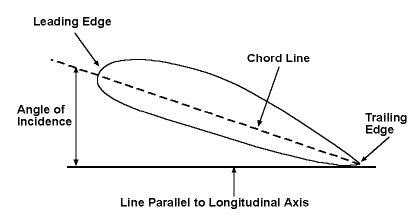Aangle of incidenceAngle of incidence of a wing.

1. In optics, the angle of incidence is the angle that a line, such as a ray of light, falling on a surface or interface makes with the normal drawn at the point of incidence. For a plane mirror, the angle of incidence equals the angle of reflection.

2. In aerodynamics, the angle of incidence is the angle at which a wing is fixed to the fuselage of an airplane. It is the angle formed by the chord of the airfoil and the longitudinal axis of the plane. This longitudinal axis is the imaginary line drawn through the fuselage from the front of the airplane to the rear.